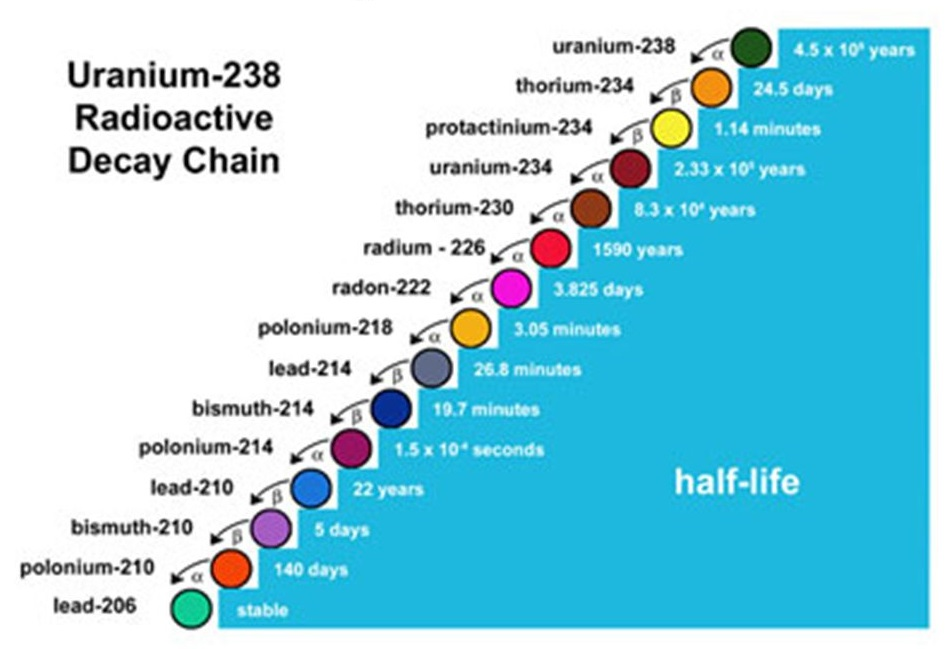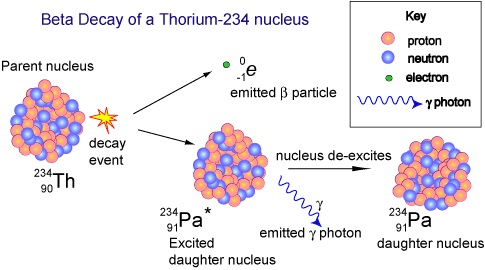# Radioactivity and Matter IRadioactive decay or ‘radioactivity’ is a physical process whereby certain unstable nuclei break up or decay spontaneously.  Radioactivity is an energetic process.

## The Valley of Stability

In Nuclear Physics parlance, the valley of stability characterises the stability of nuclides to radioactive decay, based on their binding energy.

The binding energy is the minimum energy required to disassemble an atom nucleus into its separate parts, i.e. protons and neutrons.

The valley of stability is a helpful visualisation tool for interpreting and understanding properties of nuclear decay processes, such as nuclear fission.

The shape of the valley reflects the profile of binding energy as a function of the numbers of neutrons and protons, with the lowest part of the valley corresponding to the ‘region’ where the most stable nuclei are found.  And the highest part of the slope with the most unstable radioactive nuclides.

## Radioactivity and the Decay Chain of Uranium

Radioactivity often proceeds via a sequence of steps, known as a decay chain.

For example, Uranium (238U) is the most common form of uranium found in Nature.  Over 99% of the uranium in Earth is present as this radioactive isotope.

Uranium (238U) decays to Thorium (234Th), which then decays to Protactinium (234Pa).${}^{238}_{92} U \rightarrow {}^{234}_{90} Th \rightarrow {}^{234}_{91} Pa \rightarrow {}^{234}_{92} U \rightarrow {}^{230}_{90} Th \rightarrow {}^{226}_{88} Ra \rightarrow {}^{222}_{86} Rn \rightarrow {}^{218}_{84} Po$$\rightarrow {}^{214}_{82} Pb \rightarrow {}^{214}_{83} Bi \rightarrow {}^{214}_{84} Po \rightarrow {}^{210}_{82} Pb \rightarrow {}^{210}_{83} Bi \rightarrow {}^{210}_{84} Po \rightarrow {}^{206}_{82} Pb$

And so on, and so forth… eventually reaching the stable isotope of Lead (206Pb).

## Half-Life of Radioactive Elements

You will have no doubt heard about the term “half-life” when applied to radioactive atoms.

The half-life t1/2 is the time required for a quantity to reduce to half its initial value.  It describes how quickly unstable atoms undergo, or how long stable atoms survive, radioactive decay.

However, a half-life usually describes the decay of discrete entities.  In that case, it does not work to use the definition that states “half-life is the time required for exactly half of the entities to decay”.

For example, if there is just one radioactive atom, and its half-life is one second, there will not be “half of an atom” left after one second.

A half-life period is defined in terms of probability.

### on average.

In other words, the probability of a radioactive atom decaying within its half-life is 50%.

Nevertheless, when many identical decaying atoms are concerned, the law of large numbers suggests that it is a very good approximation to say half of the atoms remain after one half-life.

# Types of Radioactivity

How dangerous radiation is really depends on the radiation type, and on how much of it is around.

The types of decay include α-decay (alpha decay), β-decay (beta minus decay) and γ-decay (gamma decay).

## α-decay

Alpha-decay is a type of radioactive decay in which an atomic nucleus emits an alpha particle.

Uranium isotopes undergo spontaneous radioactive decay into Uranium-234, by way of Thorium-234.

This type of radioactive decay in which an atomic nucleus emits an α-particle, and swiftly transforms (or ‘decays’) into an atom with a mass number 4 less and atomic number 2 less.  This “mystery” particle is the nucleus of a helium atom 42He, with mass number A = 4 and atomic number Z = 2.

The Uranium nucleus spontaneously decays into the lighter element Thorium, while releasing alpha-radiation.${}^{238}_{92} U \rightarrow {}^{234}_{90} Th + \alpha + energy$

The emitted α-particle has 2 protons and 2 neutrons, enough to make it a brand new helium nucleus, which goes on to attract 2 electrons to form a fully-fledged helium atom.${}^{238}_{92} U \rightarrow {}^{234}_{90} Th + {}^{4}_{2} He + energy$

##β–-decay

Beta-decay involves the emission of an electron e from the nucleus of an atom.The election is created by the decay, just as a photon is created when an atom makes a transition from a higher energy level to a lower energy level.

For a Thorium-234 atom, the reaction is${}^{234}_{90} Th \rightarrow {}^{234}_{91} Pa + e^- + \bar\nu_e + energy$

In the case of a Caesium-137 atom, we have the following reaction${}^{137}_{55} Cs \rightarrow {}^{137}_{56} Ba + e^- + \bar\nu_e + energy$

## γ-decay

In contrast to the processes of α- and β-decay, this type of radioactive decay involves no change in the numbers of neutrons and protons.  Gamma-decay occurs when a nucleus finds itself in an excited state.  A quantum jump down to the ground state, with the same number of neutrons and protons, is accompanied by the emission of a photon, as with transitions in atoms.

For Proactinium-234, we have${}^{234}_{91} Pa (excited \; state) \rightarrow {}^{234}_{91} Pa (ground \; state) + \gamma$

And Barium-137 gives${}^{137}_{56} Ba (excited \; state) \rightarrow {}^{137}_{56} Ba (ground \; state) + \gamma$

# Where Does the Energy Come From?

All these types of radioactive decay liberate energy.

Nuclear decay reactions always balance in the following ways:

• #### Electric charge is always conserved.

The net charge on the products of a nuclear decay is the same as the net charge of the original nucleus.

• #### Mass number is conserved.

The total number of nucleons in the products is the same as that in the original nucleus.

So where does the energy come from?

## The α-decay of Uranium-234

For example, the α-decay of U-234 liberates 4.86 MeV of kinetic energy carried away by the new particle:${}^{234}_{92} U \rightarrow {}^{230}_{90} Th + {}^{4}_{2} He + 4.86 MeV$

However, accurate measurements of the masses of the α-particle and Th-234 nucleus reveal that their sum is is less than the mass of the original nucleus of U-238 by about 8.66 x 10-30 kg.$mass \; {}^{234}_{92} U = mass \; {}^{230}_{90} Th + mass \; {}^{4}_{2} He + 8.66 \times 10^{-30} kg$

An infinitesimally small amount!

## Matter to Energy

This tiny mass was converted into energy during the radioactive decay.

Assuming a value for the speed of light of c = 3.00 x 108 ms-1 tells us that this lost mass is equivalent to an energy of$E = mc^2 = 8.66 \times 10^{-30} kg \times (3.00 \times 10^8 ms^{-1})^2 = 7.79 \times 10^{-13} J$

Thus the α-decay of one atom of U-234 liberates 7.79 x 10-13 Joules of energy, mostly carried away as kinetic energy by the α-particle.

Since$1 \; Joule = \frac{1eV}{1.60 \times 10^{-19}}$

That’s equivalent to$E = 7.79 \times 10^{-13} J \times \frac{1eV}{1.60 \times 10^{-19}} = 4.86 MeV$

Note that the amount of energy released per atom is very small.

## Little 'Bytes' about Natural Phenomena, Theoretical Physics and the Latest Worldwide Scientific Findings. Edited from Glasgow, Scotland.